SNAP Library 6.0, Developer Reference  2020-12-09 16:24:20 SNAP, a general purpose, high performance system for analysis and manipulation of large networks
TDir Class Reference

`#include <xfl.h>`

## Static Public Member Functions

static TStr GetCurDir ()

static TStr GetExeDir ()

static bool Exists (const TStr &FPathFNm)

static bool GenDir (const TStr &FPathFNm)

static bool DelDir (const TStr &FPathFNm)

## Detailed Description

Definition at line 73 of file xfl.h.

## Member Function Documentation

 bool TDir::DelDir ( const TStr & FPathFNm )
static

Definition at line 262 of file xfl.cpp.

References TStr::CStr().

262  {
263  return RemoveDirectory(FPathFNm.CStr())!=0;
264 }
char * CStr()
Definition: dt.h:479

Here is the call graph for this function: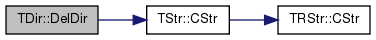bool TDir::Exists ( const TStr & FPathFNm )
static

Definition at line 249 of file xfl.cpp.

References TStr::CStr().

249  {
250 #if defined(GLib_UNIX)
251  struct stat Stat;
252  const int ErrCd = stat(FPathFNm.CStr(), &Stat);
253  if (ErrCd == 0 && S_ISDIR(Stat.st_mode)) { return true; }
254 #endif
255  return false;
256 }
char * CStr()
Definition: dt.h:479

Here is the call graph for this function: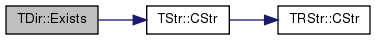bool TDir::GenDir ( const TStr & FPathFNm )
static

Definition at line 258 of file xfl.cpp.

References TStr::CStr().

258  {
259  return CreateDirectory(FPathFNm.CStr(), NULL)!=0;
260 }
char * CStr()
Definition: dt.h:479

Here is the call graph for this function: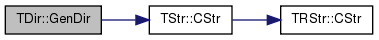TStr TDir::GetCurDir ( )
static

Definition at line 233 of file xfl.cpp.

References TStr::GetNrFPath(), and IAssert.

Referenced by TGnuPlot::CreatePlotFile(), and TStr::GetNrAbsFPath().

233  {
234  const int MxBfL=1000;
235  char Bf[MxBfL];
236  int BfL=GetCurrentDirectory(MxBfL, Bf);
237  IAssert((BfL!=0)&&(BfL<MxBfL));
238  return TStr::GetNrFPath(TStr(Bf));
239 }
#define IAssert(Cond)
Definition: bd.h:262
static TStr GetNrFPath(const TStr &FPath)
Definition: dt.cpp:1430
Definition: dt.h:412

Here is the call graph for this function: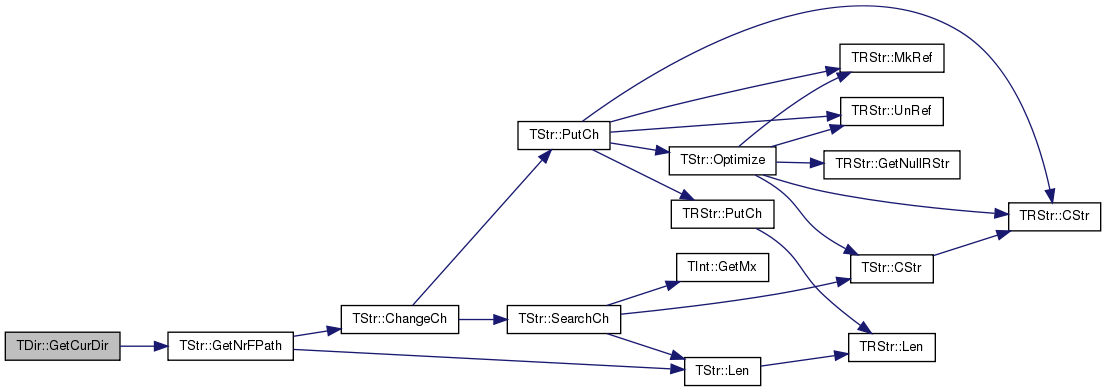Here is the caller graph for this function: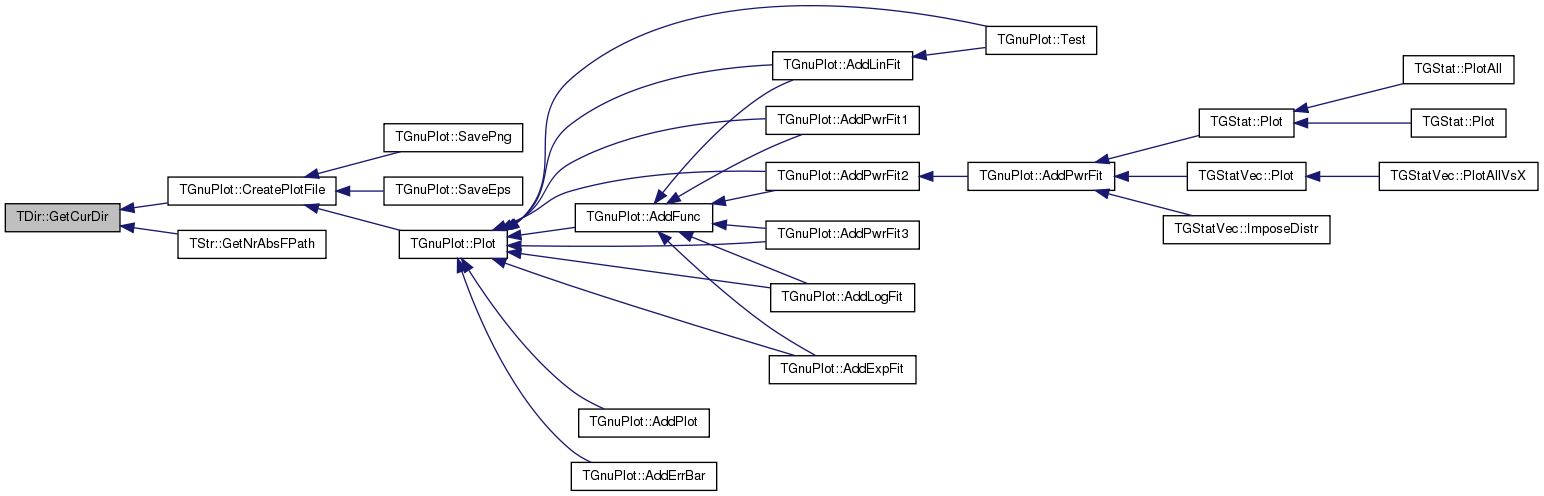TStr TDir::GetExeDir ( )
static

Definition at line 241 of file xfl.cpp.

References TStr::GetNrFPath(), and IAssert.

241  {
242  const int MxBfL=1000;
243  char Bf[MxBfL];
244  int BfL=GetModuleFileName(NULL, Bf, MxBfL);
245  IAssert((BfL!=0)&&(BfL<MxBfL));
246  return TStr::GetNrFPath(TStr(Bf).GetFPath());
247 }
#define IAssert(Cond)
Definition: bd.h:262
static TStr GetNrFPath(const TStr &FPath)
Definition: dt.cpp:1430
Definition: dt.h:412

Here is the call graph for this function: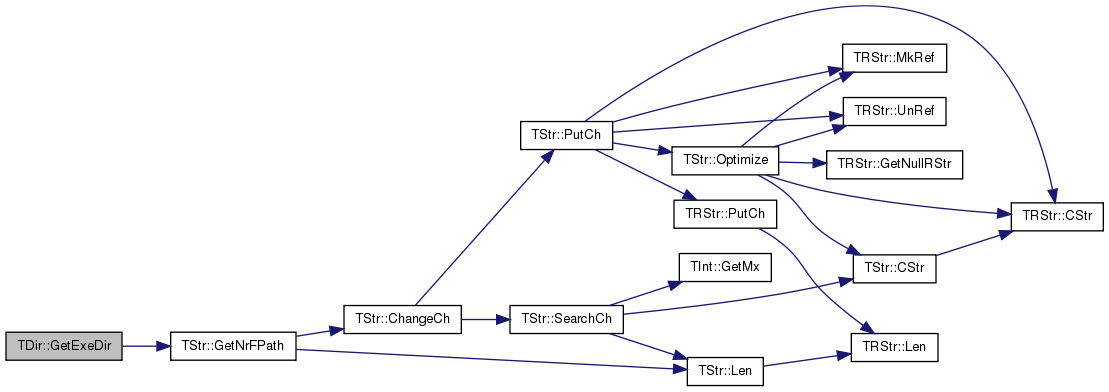The documentation for this class was generated from the following files: